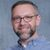Announcements
Sept. 27, 10AM ET Do more with Qlik, Insight Advisor – our intelligent AI-assistant in Qlik Sense: Register
cancel
Showing results for
Did you mean:Creator III

## Using variable from input box in expression

Hi

I have an input box where I pick values from a list for variable vMargin.

I now want to use this variable in a simple expression in a chart, I'm getting a null value.

If i just use the expression =\$(vMargin), I get the value of variable as picked in the input box.

However, when I add another element to the margin, even a constant e.g. 100/\$(vMargin), I'm getting a null value.

Any ideas?

Thanks

Joe

1 Solution

Accepted SolutionsContributor III

I was able to get this to work using sample data if this is what you were going for:

sum({<Dim={'ValueA'}>} fact)/(1-\$(vMargin))

5 RepliesContributor III

try adding sum or aggr such as sum(100/\$(vMargin)) or aggr(100/\$(vMargin), Dim)Creator III
Author

Thanks Justin

The actual expression I have uses a Set Analysis expression as the numerator, and then the variable as the denominator.

So Sum({<Dim={'ValueA'}>}/(1-\$(vMargin))Contributor III

I was able to get this to work using sample data if this is what you were going for:

sum({<Dim={'ValueA'}>} fact)/(1-\$(vMargin))Creator III
Author

Thanks Justin

That works fine for me now.

Regards

JoeContributor III